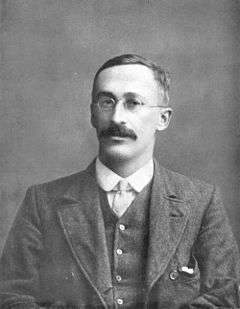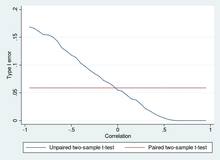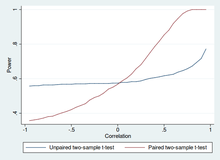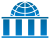# Student's t-test

A t-test is any statistical hypothesis test in which the test statistic follows a Student's t-distribution under the null hypothesis. It can be used to determine if two sets of data are significantly different from each other.

A t-test is most commonly applied when the test statistic would follow a normal distribution if the value of a scaling term in the test statistic were known. When the scaling term is unknown and is replaced by an estimate based on the data, the test statistics (under certain conditions) follow a Student's t distribution.

## HistoryWilliam Sealy Gosset, who developed the "t-statistic" and published it under the pseudonym of "Student".

The t-statistic was introduced in 1908 by William Sealy Gosset, a chemist working for the Guinness brewery in Dublin, Ireland ("Student" was his pen name).

Gosset had been hired due to Claude Guinness's policy of recruiting the best graduates from Oxford and Cambridge to apply biochemistry and statistics to Guinness's industrial processes. Gosset devised the t-test as an economical way to monitor the quality of stout. The Student's t-test work was submitted to and accepted in the journal Biometrika and published in 1908. Company policy at Guinness forbade its chemists from publishing their findings, so Gosset published his statistical work under the pseudonym "Student" (see Student's t-distribution for a detailed history of this pseudonym, which is not to be confused with the literal term, "student").

Guinness had a policy of allowing technical staff leave for study (so-called "study leave"), which Gosset used during the first two terms of the 1906–1907 academic year in Professor Karl Pearson's Biometric Laboratory at University College London. Gosset's identity was then known to fellow statisticians and to editor-in-chief Karl Pearson.

It is not clear how much of the work Gosset performed while he was at Guinness and how much was done when he was on study leave at University College London.

## Uses

Among the most frequently used t-tests are:

• A one-sample location test of whether the mean of a population has a value specified in a null hypothesis.
• A two-sample location test of the null hypothesis such that the means of two populations are equal. All such tests are usually called Student's t-tests, though strictly speaking that name should only be used if the variances of the two populations are also assumed to be equal; the form of the test used when this assumption is dropped is sometimes called Welch's t-test. These tests are often referred to as "unpaired" or "independent samples" t-tests, as they are typically applied when the statistical units underlying the two samples being compared are non-overlapping.
• A test of the null hypothesis that the difference between two responses measured on the same statistical unit has a mean value of zero. For example, suppose we measure the size of a cancer patient's tumor before and after a treatment. If the treatment is effective, we expect the tumor size for many of the patients to be smaller following the treatment. This is often referred to as the "paired" or "repeated measures" t-test: see paired difference test.
• A test of whether the slope of a regression line differs significantly from 0.

## Assumptions

Most t-test statistics have the form t = Z/s, where Z and s are functions of the data. Typically, Z is designed to be sensitive to the alternative hypothesis (i.e., its magnitude tends to be larger when the alternative hypothesis is true), whereas s is a scaling parameter that allows the distribution of t to be determined.

As an example, in the one-sample t-test, whereis the sample mean from a sample X1,X2,,Xn, of size n, s is the ratio of sample standard deviation over population standard deviation, σ is the population standard deviation of the data, and μ is the population mean.

The assumptions underlying a t-test are that

In a specific type of t-test, these conditions are consequences of the population being studied, and of the way in which the data are sampled. For example, in the t-test comparing the means of two independent samples, the following assumptions should be met:

• Each of the two populations being compared should follow a normal distribution. This can be tested using a normality test, such as the Shapiro–Wilk or Kolmogorov–Smirnov test, or it can be assessed graphically using a normal quantile plot.
• If using Student's original definition of the t-test, the two populations being compared should have the same variance (testable using F-test, Levene's test, Bartlett's test, or the Brown–Forsythe test; or assessable graphically using a Q–Q plot). If the sample sizes in the two groups being compared are equal, Student's original t-test is highly robust to the presence of unequal variances. Welch's t-test is insensitive to equality of the variances regardless of whether the sample sizes are similar.
• The data used to carry out the test should be sampled independently from the two populations being compared. This is in general not testable from the data, but if the data are known to be dependently sampled (i.e., if they were sampled in clusters), then the classical t-tests discussed here may give misleading results.

Most two-sample t-tests are robust to all but large deviations from the assumptions.

## Unpaired and paired two-sample t-testsType I error of unpaired and paired two-sample t-tests as a function of the correlation. The simulated random numbers originate from a bivariate normal distribution with a variance of 1. The significance level is 5% and the number of cases is 60.Power of unpaired and paired two-sample t-tests as a function of the correlation. The simulated random numbers originate from a bivariate normal distribution with a variance of 1 and a deviation of the expected value of 0.4. The significance level is 5% and the number of cases is 60.

Two-sample t-tests for a difference in mean involve independent samples or unpaired samples. Paired t-tests are a form of blocking, and have greater power than unpaired tests when the paired units are similar with respect to "noise factors" that are independent of membership in the two groups being compared. In a different context, paired t-tests can be used to reduce the effects of confounding factors in an observational study.

### Independent (unpaired) samples

The independent samples t-test is used when two separate sets of independent and identically distributed samples are obtained, one from each of the two populations being compared. For example, suppose we are evaluating the effect of a medical treatment, and we enroll 100 subjects into our study, then randomly assign 50 subjects to the treatment group and 50 subjects to the control group. In this case, we have two independent samples and would use the unpaired form of the t-test. The randomization is not essential here – if we contacted 100 people by phone and obtained each person's age and gender, and then used a two-sample t-test to see whether the mean ages differ by gender, this would also be an independent samples t-test, even though the data is observational.

### Paired samples

Paired samples t-tests typically consist of a sample of matched pairs of similar units, or one group of units that has been tested twice (a "repeated measures" t-test).

A typical example of the repeated measures t-test would be where subjects are tested prior to a treatment, say for high blood pressure, and the same subjects are tested again after treatment with a blood-pressure lowering medication. By comparing the same patient's numbers before and after treatment, we are effectively using each patient as their own control. That way the correct rejection of the null hypothesis (here: of no difference made by the treatment) can become much more likely, with statistical power increasing simply because the random between-patient variation has now been eliminated. Note however that an increase of statistical power comes at a price: more tests are required, each subject having to be tested twice. Because half of the sample now depends on the other half, the paired version of Student's t-test has only "n/2–1" degrees of freedom (with n being the total number of observations). Pairs become individual test units, and the sample has to be doubled to achieve the same number of degrees of freedom.

A paired samples t-test based on a "matched-pairs sample" results from an unpaired sample that is subsequently used to form a paired sample, by using additional variables that were measured along with the variable of interest. The matching is carried out by identifying pairs of values consisting of one observation from each of the two samples, where the pair is similar in terms of other measured variables. This approach is sometimes used in observational studies to reduce or eliminate the effects of confounding factors.

Paired samples t-tests are often referred to as "dependent samples t-tests".

## Calculations

Explicit expressions that can be used to carry out various t-tests are given below. In each case, the formula for a test statistic that either exactly follows or closely approximates a t-distribution under the null hypothesis is given. Also, the appropriate degrees of freedom are given in each case. Each of these statistics can be used to carry out either a one-tailed or two-tailed test.

Once the t value and degrees of freedom are determined, a p-value can be found using a table of values from Student's t-distribution. If the calculated p-value is below the threshold chosen for statistical significance (usually the 0.10, the 0.05, or 0.01 level), then the null hypothesis is rejected in favor of the alternative hypothesis.

### One-sample t-test

In testing the null hypothesis that the population mean is equal to a specified value μ0, one uses the statisticwhereis the sample mean, s is the sample standard deviation of the sample and n is the sample size. The degrees of freedom used in this test are n  1. Although the parent population does not need to be normally distributed, the distribution of the population of sample means,, is assumed to be normal. By the central limit theorem, if the sampling of the parent population is independent then the sample means will be approximately normal. (The degree of approximation will depend on how close the parent population is to a normal distribution and the sample size, n.)

### Slope of a regression line

Suppose one is fitting the modelwhere x is known, α and β are unknown, and ε is a normally distributed random variable with mean 0 and unknown variance σ2, and Y is the outcome of interest. We want to test the null hypothesis that the slope β is equal to some specified value β0 (often taken to be 0, in which case the null hypothesis is that x and y are independent).

LetThenhas a t-distribution with n  2 degrees of freedom if the null hypothesis is true. The standard error of the slope coefficient:can be written in terms of the residuals. LetThenis given by:### Independent two-sample t-test

#### Equal sample sizes, equal variance

Given two groups (1, 2), this test is only applicable when:

• the two sample sizes (that is, the number, n, of participants of each group) are equal;
• it can be assumed that the two distributions have the same variance;

Violations of these assumptions are discussed below.

The t statistic to test whether the means are different can be calculated as follows:whereHereis the pooled standard deviation for n=n1=n2 andandare the unbiased estimators of the variances of the two samples. The denominator of t is the standard error of the difference between two means.

For significance testing, the degrees of freedom for this test is 2n  2 where n is the number of participants in each group.

#### Equal or unequal sample sizes, equal variance

This test is used only when it can be assumed that the two distributions have the same variance. (When this assumption is violated, see below.) Note that the previous formulae are a special case valid when both samples have equal sizes: n = n1 = n2. The t statistic to test whether the means are different can be calculated as follows:whereis an estimator of the pooled standard deviation of the two samples: it is defined in this way so that its square is an unbiased estimator of the common variance whether or not the population means are the same. In these formulae, ni − 1 is the number of degrees of freedom for each group, and the total sample size minus two (that is, n1 + n2 − 2) is the total number of degrees of freedom, which is used in significance testing.

#### Equal or unequal sample sizes, unequal variances

Main article: Welch's t-test

This test, also known as Welch's t-test, is used only when the two population variances are not assumed to be equal (the two sample sizes may or may not be equal) and hence must be estimated separately. The t statistic to test whether the population means are different is calculated as:whereHere s2i is the unbiased estimator of the variance of each of the two samples with ni = number of participants in group i, i=1 or 2. Note that in this caseis not a pooled variance. For use in significance testing, the distribution of the test statistic is approximated as an ordinary Student's t distribution with the degrees of freedom calculated usingThis is known as the Welch–Satterthwaite equation. The true distribution of the test statistic actually depends (slightly) on the two unknown population variances (see Behrens–Fisher problem).

### Dependent t-test for paired samples

This test is used when the samples are dependent; that is, when there is only one sample that has been tested twice (repeated measures) or when there are two samples that have been matched or "paired". This is an example of a paired difference test.For this equation, the differences between all pairs must be calculated. The pairs are either one person's pre-test and post-test scores or between pairs of persons matched into meaningful groups (for instance drawn from the same family or age group: see table). The average (XD) and standard deviation (sD) of those differences are used in the equation. The constant μ0 is non-zero if you want to test whether the average of the difference is significantly different from μ0. The degree of freedom used is n  1, where n represents the number of pairs.

Example of repeated measures
Number Name Test 1 Test 2
1 Mike 35% 67%
2 Melanie 50% 46%
3 Melissa 90% 86%
4 Mitchell 78% 91%
Example of matched pairs
Pair Name Age Test
1 John 35 250
1 Jane 36 340
2 Jimmy 22 460
2 Jessy 21 200

## Worked examples

Let A1 denote a set obtained by drawing a random sample of six measurements:and let A2 denote a second set obtained similarly:These could be, for example, the weights of screws that were chosen out of a bucket.

We will carry out tests of the null hypothesis that the means of the populations from which the two samples were taken are equal.

The difference between the two sample means, each denoted by, which appears in the numerator for all the two-sample testing approaches discussed above, isThe sample standard deviations for the two samples are approximately 0.05 and 0.12, respectively. For such small samples, a test of equality between the two population variances would not be very powerful. Since the sample sizes are equal, the two forms of the two-sample t-test will perform similarly in this example.

### Unequal variances

If the approach for unequal variances (discussed above) is followed, the results areand the degrees of freedom, df,The test statistic is approximately 1.959, which gives a 2-tailed test p-value of 0.09077.

### Equal variances

If the approach for equal variances (discussed above) is followed, the results areand the degrees of freedom, df,The test statistic is approximately equal to 1.959, which gives 2-sided p-value of 0.07857.

## Alternatives to the t-test for location problems

The t-test provides an exact test for the equality of the means of two normal populations with unknown, but equal, variances. (The Welch's t-test is a nearly exact test for the case where the data are normal but the variances may differ.) For moderately large samples and a one tailed test, the t is relatively robust to moderate violations of the normality assumption.

For exactness, the t-test and Z-test require normality of the sample means, and the t-test additionally requires that the sample variance follows a scaled χ2 distribution, and that the sample mean and sample variance be statistically independent. Normality of the individual data values is not required if these conditions are met. By the central limit theorem, sample means of moderately large samples are often well-approximated by a normal distribution even if the data are not normally distributed. For non-normal data, the distribution of the sample variance may deviate substantially from a χ2 distribution. However, if the sample size is large, Slutsky's theorem implies that the distribution of the sample variance has little effect on the distribution of the test statistic. If the data are substantially non-normal and the sample size is small, the t-test can give misleading results. See Location test for Gaussian scale mixture distributions for some theory related to one particular family of non-normal distributions.

When the normality assumption does not hold, a non-parametric alternative to the t-test can often have better statistical power. For example, for two independent samples when the data distributions are asymmetric (that is, the distributions are skewed) or the distributions have large tails, then the Wilcoxon rank-sum test (also known as the Mann–Whitney U test) can have three to four times higher power than the t-test. The nonparametric counterpart to the paired samples t-test is the Wilcoxon signed-rank test for paired samples. For a discussion on choosing between the t-test and nonparametric alternatives, see Sawilowsky (2005).

One-way analysis of variance (ANOVA) generalizes the two-sample t-test when the data belong to more than two groups.

## Multivariate testing

A generalization of Student's t statistic, called Hotelling's t-squared statistic, allows for the testing of hypotheses on multiple (often correlated) measures within the same sample. For instance, a researcher might submit a number of subjects to a personality test consisting of multiple personality scales (e.g. the Minnesota Multiphasic Personality Inventory). Because measures of this type are usually positively correlated, it is not advisable to conduct separate univariate t-tests to test hypotheses, as these would neglect the covariance among measures and inflate the chance of falsely rejecting at least one hypothesis (Type I error). In this case a single multivariate test is preferable for hypothesis testing. Fisher's Method for combining multiple tests with alpha reduced for positive correlation among tests is one. Another is Hotelling's T 2 statistic follows a T 2 distribution. However, in practice the distribution is rarely used, since tabulated values for T 2 are hard to find. Usually, T 2 is converted instead to an F statistic.

For a one-sample multivariate test, the hypothesis is that the mean vector () is equal to a given vector (). The test statistic is Hotelling's t 2:where n is the sample size,is the vector of column means andis asample covariance matrix.

For a two-sample multivariate test, the hypothesis is that the mean vectors (,) of two samples are equal. The test statistic is Hotelling's two-sample t 2:## Software implementations

Many spreadsheet programs and statistics packages, such as QtiPlot, LibreOffice Calc, Microsoft Excel, SAS, SPSS, Stata, DAP, gretl, R, Python, PSPP, Matlab and Minitab, include implementations of Student's t-test.

Language/Program Function Notes
Microsoft Excel pre 2010 TTEST(array1, array2, tails, type) See
Microsoft Excel 2010 and later T.TEST(array1, array2, tails, type) See
LibreOffice TTEST(Data1; Data2; Mode; Type) See
Google Sheets TTEST(range1, range2, tails, type) See
Python scipy.stats.ttest_ind(a, b, axis=0, equal_var=True) See
Matlab ttest(data1, data2) See
Mathematica TTest[{data1,data2}] See
R t.test(data1, data2, var.equal=TRUE) See
SAS PROC TTEST See
Java tTest(sample1, sample2) See
Julia EqualVarianceTTest(sample1, sample2) See
Stata ttest data1 == data2 See

1. Richard Mankiewicz (2004). The Story of Mathematics (Paperback ed.). Princeton, NJ: Princeton University Press. p. 158. ISBN 9780691120461.
2. Fisher Box, Joan (1987). "Guinness, Gosset, Fisher, and Small Samples". Statistical Science. 2 (1): 45–52. doi:10.1214/ss/1177013437. JSTOR 2245613.
3. http://www.aliquote.org/cours/2012_biomed/biblio/Student1908.pdf
4. "The Probable Error of a Mean" (PDF). Biometrika. 6 (1): 1–25. 1908. Retrieved 24 July 2016.
5. Raju, T. N. (2005). "William Sealy Gosset and William A. Silverman: Two "students" of science". Pediatrics. 116 (3): 732–5. doi:10.1542/peds.2005-1134. PMID 16140715.
6. Dodge, Yadolah (2008). The Concise Encyclopedia of Statistics. Springer Science & Business Media. pp. 234–5. ISBN 978-0-387-31742-7.
7. Fadem, Barbara (2008). High-Yield Behavioral Science (High-Yield Series). Hagerstwon, MD: Lippincott Williams & Wilkins. ISBN 0-7817-8258-9.
8. Zimmerman, Donald W. (1997). "A Note on Interpretation of the Paired-Samples t Test". Journal of Educational and Behavioral Statistics. 22 (3): 349–360. doi:10.3102/10769986022003349. JSTOR 1165289.
9. Markowski, Carol A.; Markowski, Edward P. (1990). "Conditions for the Effectiveness of a Preliminary Test of Variance". The American Statistician. 44 (4): 322–326. doi:10.2307/2684360. JSTOR 2684360.
10. Martin Bland (1995). An Introduction to Medical Statistics. Oxford University Press. p. 168. ISBN 978-0-19-262428-4.
11. John A. Rice (2006), Mathematical Statistics and Data Analysis, Third Edition, Duxbury Advanced.
12. David, H. A.; Gunnink, Jason L. (1997). "The Paired t Test Under Artificial Pairing". The American Statistician. 51 (1): 9–12. doi:10.2307/2684684. JSTOR 2684684.
13. George Box, William Hunter, and J. Stuart Hunter, Statistics for Experimenters, ISBN 978-0471093152, pp. 66–67.
14. Sawilowsky, Shlomo S.; Blair, R. Clifford (1992). "A More Realistic Look at the Robustness and Type II Error Properties of the t Test to Departures From Population Normality". Psychological Bulletin. 111 (2): 352–360. doi:10.1037/0033-2909.111.2.352.
15. Blair, R. Clifford; Higgins, James J. (1980). "A Comparison of the Power of Wilcoxon's Rank-Sum Statistic to That of Student's t Statistic Under Various Nonnormal Distributions". Journal of Educational Statistics. 5 (4): 309–335. doi:10.2307/1164905. JSTOR 1164905.
16. Fay, Michael P.; Proschan, Michael A. (2010). "Wilcoxon–Mann–Whitney or t-test? On assumptions for hypothesis tests and multiple interpretations of decision rules". Statistics Surveys. 4: 1–39. doi:10.1214/09-SS051. PMC 2857732. PMID 20414472.
17. Sawilowsky, Shlomo S. (2005). "Misconceptions Leading to Choosing the t Test Over The Wilcoxon Mann–Whitney Test for Shift in Location Parameter". Journal of Modern Applied Statistical Methods. 4 (2): 598–600. Retrieved 2014-06-18.
• Boneau, C. Alan (1960). "The effects of violations of assumptions underlying the t test". Psychological Bulletin. 57 (1): 49–64. doi:10.1037/h0041412.
• Edgell, Stephen E., & Noon, Sheila M (1984). "Effect of violation of normality on the t test of the correlation coefficient". Psychological Bulletin. 95 (3): 576–583. doi:10.1037/0033-2909.95.3.576.Wikiversity has learning materials about t-test